Set Theory Symbols

Sets Math - Symbols Used in Set Theory

A set is defined by a collection of objects. The objects of a particular set are its elements. Set theory is the study of such sets, and the relation that connects the sets to its elements. Set Theory was first created by Sir Georg Ferdinand Ludwig Philipp Cantor who was a German mathematician. The history and creation of set theory is quite different from the history of other areas of mathematics. It was created to be able to talk about collections of objects that represent a particular set. Set theory has turned out to be an extremely important tool for defining some of the most complex and significant mathematical structures.

Some of the basic examples of set:

• • The collection of all green bottles.

• • The collection of negative numbers.

• • The collection of people born before 1995.

• • The collection of greatest football players.

• All of the above collections are sets. However, the collection of greatest football players is not well-defined. Usually, we restrict our focus to just well-defined sets.

How to define sets?

There are three ways in which you define sets:

• • Descriptive form

• • Roster(listing) form

• • Set builder form

• For example: Define/represent a set of odd natural numbers that lies between the interval of 2 to 12 in descriptive, roster and set builder form.

Descriptive form
Example: The set of all odd counting numbers between 2 and 12.

Roster (listing) form
Example: {3, 5, 7, 9, 11}

Set builder form
Example: {x | x is a natural number, x is odd, and 2< x < 12}

Note:
• 1. Use curly braces to represent sets.

• 2. Use commas to separate set elements from each other.

• 3. The variable in the set–builder notation doesn’t necessarily have to be x.

• 4. The ellipses (. . .) are used to indicate a continuation of a pattern/ series established before the ellipses i.e. {1, 2, 3, 4 . . . 100}.

• 5. The symbol | is called “such that”.

• Set Membership

An element or member of a set is also known as an object of the set.

The symbol ‘∈’ means “is an element of”.

The meaning of the symbol ∈ “is not an element of”.

Capital letters are used to denote sets and lowercase letters are used to represent its objects i.e. A = {2, 4, 6, 8}. Thus, a means x is an element of A.

Is 2 ∈ {2, 4,6, 8}? The answer is yes.

Is 2 ∈ {1, 3, 5, 7, 11}? The answer is no.

Important Sets to remember

• • N denotes Natural or Counting numbers: {1, 2, 3 . .}

• • W denotes Whole Numbers: {0, 1, 2, 3 . . .}

• • I denote Integers: {. ., -5, -4, -3, -2, -1, 0, 1, 2, 3 . .}

• • Q denotes Rational numbers: {p/q | p, q ∈ I, q 6= 0}.

• • R denotes Real Numbers: {x | x is a number that can be written as a decimal}.

• • Irrational numbers: {x | x is a real number and it cannot be written as a quotient of integers}

• • Examples are: π, √ 3, and 3√ 9.

• • ∅ represents Empty Set: {}, the set that contains nothing.

• • U represents Universal Set: the set of all objects currently under discussion.

• NOTE: Any rational number can be written either as a terminating decimal (e.g 0.5, 0.333, or 0.8578966) or as a repeating decimal (e.g 0.333 or 123.392545).

The decimal digits of an irrational number never terminates and never repeats.

The set {∅} is not empty but is a set that contains the empty set.

Question:
Is ∅∈ {a, b, c}?
Is ∅∈ {∅, {∅}}?
Is ∅∈ {{∅}}?
Is 1/3 ∈ {x | x = 1/p, p ∈ N}?

Cardinality

The cardinality of a set means the number of unique elements within the set.

The symbol of cardinality is n (A) or |A|, where A is a set.

If the cardinality of a set is a whole number, then it is called as a finite set.

If a set is so large that its cardinality is uncountable, then it is called as an infinite set.

Note: we don’t need to consider about the order or how many times an object is included in a particular set. Thus, {3, 1, 2, 4}, {2, 3, 4, 1}, and {1, 2, 2, 3, 3, 3, 4, 4, 4, 4} all describe the same set.

Question:
If A = {5, 7, 9, 11, 13}
B = {5, 10, 15 . . . 100}
C = {3, 5, 7, 9, . . .}
D = {1, 3, 3, 1, 2}
P = {x | x is odd, and x < 12}

Find:
n (A) =?
n (B) =?
n(C) =?
n (D) =?
n (P) =?

Set Equality

The sets P and Q are equal (written P = Q), if and only if:

• i. Every element of P is an element of Q,

• ii. Every element of Q is an element of P. In other words, set P and Q are equal if and only if they contain exactly the same elements

• {x, y, z} = {z, x, y} = {z, y, y, y, x, x,}
{3} = {x | x ∈ N and 1 < x < 5}?
{x | x ∈ N and x < 0} = {y | y ∈ Q and y is irrational}

Venn Diagrams & Subsets

Universe: It is the set consisting of all elements under consideration for a particular situation. It is called the universal set and is denoted by ‘ꓴ’. Venn diagrams are used to represent sets and their relationships with each other. Here, X is a universal set represented by a rectangle. Sets are represented by shaded region, circles or other shapes within the rectangle.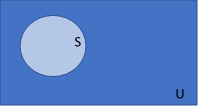Complement of a Set

If a set contains some specific objects, then complement of that will not contain those elements or objects. For example: If P= {1, 2, 3, 4, 5, 6, 7, 8, 9, 10}, A= {1, 2, 3, 4, 5}, then A’= {6, 7, 8, 9, 10}.

Question

Let Q = {1, 2, 3 . . . 8}, P = {1, 2, 5, 6}, and R = {2, 4, 5, 7, 8}
What is: P’, the complement of P?
What is: R’, the complement of R?
What is: Q’, the complement of Q?
What is: ∅’, the complement of ∅?

Subset

Set X is a subset of set Y if every element of X is also an element of Y and in other words, Y contains all of the elements of X which is denoted X ⊆ Y.

R, S, and T are the sets that are below in the Venn diagram below, which are subsets: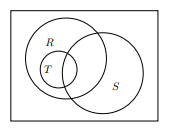If U = {2, 3, 4 . . . 8}, R = {2, 5, 6}, S = {2, 4, 5, 7, 8}, and T = {2, 6}. What element(s) are in the area where all the sets get overlapped? What object(s) are in the area outside all the sets?Set Equality and Proper Subsets

Sets P and Q are equal only if:

• i. P ⊆ Q and

• ii. Q ⊆ P

• Proper Subset: P ⊂ Q if P ⊆ Q and P = Q

Questions

Whether or Not a Subset?
Is the set on the left a subset of the set on the right?
• a. {a, b, c} {a, c, d, f}

• b. {a, b, c} {c, a, b}

• c. {a, b, c} {a, b, c}

• d. {a} {a, b, c}

• e. {a, c} {a, b, c, d}

• f. {a, c} {a, b, d, e, f}

• g. X X

• h. ∅ {a, b, c}

• i. ∅ ∅

• Points to remember:
• • Any set is a subset of itself and also a subset of the universal set.

• • The empty set is a subset of all the sets as well as itself.

• Questions:
Is the set on the left equal to, a proper subset of, or not a subset of the set on the right?
{1, 2, 3} I
{a, b} {a}
{a} {a, b}
{a, b, c} {a, d, e, g}
{a, b, c} {a, a, c, b, c}
{∅} {a, b, c}
{∅} {}

Power Set

S (A) is the set of all possible subsets of the set A. For example, if A = {0, 2}, then S (A) = {∅, {0}, {2}, {0, 2}}.

Find the power sets of the following and their cardinality.
S (∅) =
S ({a}) =
S ({a, b}) =
S ({a, b, c}) =

Is there a pattern?

Alternate Method of Generating Power Sets:

Under this method, a tree diagram will be used to generate S (A). Every element in the set is either in a particular subset, or it’s not.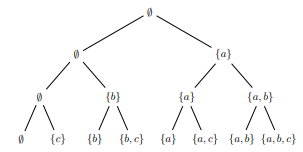The number of subsets of a set with cardinality n can be calculated by using the formula: 2 n.

The number of proper subsets can be calculated by using the formula: 2 n – 1.

Set Operations

Intersection

The intersection of two sets, X ∩ Y, is the set of elements common to both the sets, that is, X and Y: X ∩ Y = {XX ∈ X and x ∈ Y}.
Therefore, for an object to be in X ∩ Y, it must also be a member of both the sets, X and Y.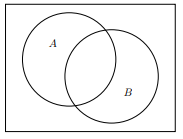Disjoint sets

Two sets which have no common elements in them are called disjoint sets. Their intersection is empty: X ∩ Y = ∅

Set Union

The union of two sets, X ∪ Y, is the set of elements belonging to either of the sets:
X ∪ Y = {x|x ∈ X or x ∈ Y}. Therefore, for an object to be in X ∪ Y, it must be a member of either X or Y. The total shaded area covered by X and Y falls under XUY.Set Difference

The difference of two sets, X − Y, is the set of elements belonging to set X and not to set Y: X − Y = {x|x∈ A and x ∈ B}Note: Generally, X − Y = Y – X.
Also, X ∈ Y means x ∈ Y’. Thus, X − Y = {x|x∈ X and x ∈ Y’} = X ∩ Y’

Questions:

Find the Unions:
{a, b, c}∪{b, f, g} =
{a, b, c}∪{a, b, c} =

Find the set difference:
{ 5, 2, 3, 1, 4} − {2, 4, 6} =
{ 1, 3, 5} {2, 6, 4} − {1, 2, 3, 4, 5} =

Given the sets:
U = {1, 2, 3, 4, 5, 6, 9}
P = {1, 2, 3, 4}
Q = {2, 4, 6}
R = {1, 3, 6, 9}

Find each of these sets:
P ∪ Q =
P ∩ Q =
P ∩ U =
P ∪ U=
P’ =
P' ∩ Q =
P’ ∪ Q =
P ∪ Q ∪ R =
P ∩ Q ∩ R =
P’ ∪ Q’ =
P’ ∩ Q’ =
P ∩ (Q∪R) =
(P’∪R) ∩ Q =
P − Q =
Q − P =
(P − Q) ∪ R’ =

In case if U = {q, r, s, t, u, v, w, x, y, z}, A = {r, s, t, i, v}, and B = {t, v, x}
Fill up the Venn diagram to represent U, A, and BShade the area in the Diagram for: A’ ∩ B’ ∩ C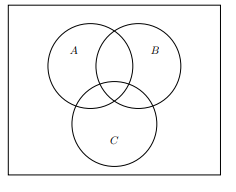Shade the area in the Diagram
• a. (A ∩ B)’

•• b. A’ ∪ B’

•Write whether the Following Sets are Disjoint?
{c, b, a} and {d, e, f, g} …
{a, b, c} and {a, b, c} …
{a, b, c} and {a, b, z} …
{a, b, c} and {x, y, z} …
{a, b, c} and ∅ …
For any A, A and ∅ …
For any A, A and A’ …
De Morgan’s Laws
For any sets P and Q,
(P ∩ Q)’ = P’ ∪ Q’
(P ∪ Q)’ = P’ ∩ Q’